# Parameter, method of variation of the

A method for approximately solving non-linear (and linear) functional and operator equations,,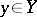, in Banach spaces, and also for qualitatively investigating them. The method of variation of the parameter consists in the following: The equation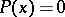, where the operatoris continuously Fréchet-differentiable up to the required order (cf. Fréchet derivative), or a certain non-linear functional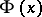connected with the solution of this equation, is generalized by introducing an auxiliary numerical (or, in general, functional) parametertaking values in a finite or infinite intervalto, where,, is an operator with values insuch thatis obtained for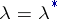:, and the equation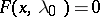is either easy to solve or a solutionof it is known. Here it is assumed thatis continuously Fréchet-differentiable inand, that is, the partial derivativesandexist and are continuous, and that the operatorfromintoexists and is continuous. To construct a solution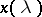of the equation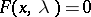on the whole interval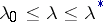one sets up the corresponding differential problem (Cauchy problem) under the assumption thatis a continuously-differentiable function with values in, defined by the equation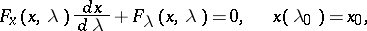(1)

or(2)

The intervalis partitioned by points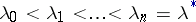into finer subintervals of lengths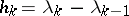,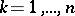, and to the Cauchy problem (2) or (1) one applies a method of numerical integration of ordinary differential equations with step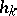(or several such methods). As a result, for constructing a solutionofone obtains methods of variation of the parameter of corresponding types. The resulting value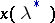is a solution of.

The solution at every step of linear problems in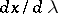of the form (1), the inversion of the linear operators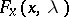in (2) or the successive approximation of the inverse operation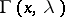proceed by various methods or once again by variation of the parameter.

The stepsare chosen by various means, for example, from the condition of minimizing the norm of the discrepancy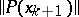as a function of, generally speaking, several variables. Here a joint choice ofand the free parameters of a method of numerical integration is also effective, for example, the Runge–Kutta method of order of accuracy, the use of the roots of Chebyshev and related polynomials, etc.

The Cauchy problem (2) is not only a means for determining an approximate solution of the equation in question, but also for proving the existence of the solution itself. A number of distinct ways of introducing the parameterhave been studied. As the numerical parameterone may also use one of the natural parameters inherent in the problem.

Depending on the way of introducing, the method of variation of the parameter is a direct or an iterative method. A joint application of a direct and an iterative method is said to give a combined method. For example, the iterative method of improved Euler–Cauchy type with step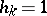(for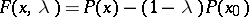,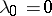and) is of the third order of accuracy and has the following form: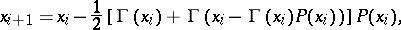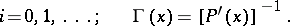Every method of numerical integration gives rise to an iterative method of variation of the parameter of a higher order of accuracy, and without the need to bring in the derivatives ofof an order higher than the first.

The use of methods of numerical integration in direct methods of variation of the parameter in conjunction with a correction of the results after each step by an iterative method of variation of the parameter (a combined method) is one of the most effective methods for solving non-linear equations.

For a broad class of problems the method of variation of the parameter has been worked-out sufficiently well. Originally it was proposed for systems of algebraic and transcendental equations, integral equations, ordinary and partial differential equations, and later for the solution of more general non-linear and operator equations. Conditions have been studied under which the solvability of the equationis guaranteed, as well as the possibility of constructing a solution of it by integrating the Cauchy problem (2) over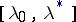and establishing the domains of its location. Convergence conditions and error estimates have also been studied, as well as problems of applying a method of variation of the parameter for the inversion and pseudo-inversion of linear operators, for the construction of pseudo-solutions (and solutions) of linear functional equations with minimal deviation in norm (in a given space) from the initial value, for the summation of operator series and the construction of certain classes of projections, for the determination of initial approximations for iteration processes, for the solution of operator differential equations and problems in linear algebra, or for proving the solvability of non-linear systems connected with variational problems and the construction of solutions of them, for the minimization of functionals and in many others. Other research concerns extensive classes of effective modifications of the method of variation of the parameter, among them successive approximation of the inverse operatoror. Also, broad classes of branching problems and non-linear problems on eigen values have been studied.(However, the case of branching may be blocked by other ways of introducing a parameteror of an additional parameter.) The method of variation of the parameter has also been treated as a method of "gradient" type, and also without the assumption that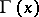exists.